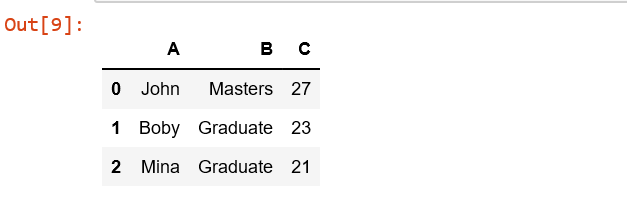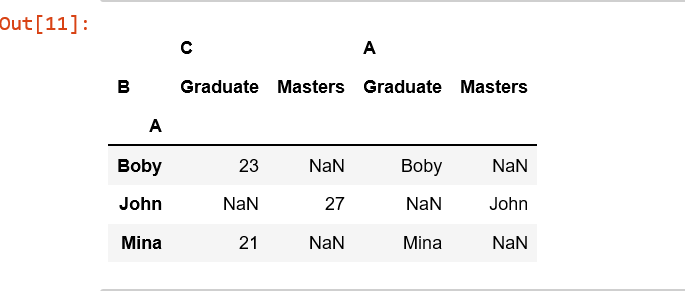# Python | Pandas.pivot()

pandas.pivot(index, columns, values) function produces pivot table based on 3 columns of the DataFrame. Uses unique values from index / columns and fills with values.

Parameters:
index[ndarray] : Labels to use to make new frame’s index
columns[ndarray] : Labels to use to make new frame’s columns
values[ndarray] : Values to use for populating new frame’s values

Returns: Reshaped DataFrame
Exception: ValueError raised if there are any duplicates.

Code:

 `# Create a simple dataframe ` ` `  `# importing pandas as pd ` `import` `pandas as pd ` ` `  `# creating a dataframe ` `df ``=` `pd.DataFrame({``'A'``: [``'John'``, ``'Boby'``, ``'Mina'``], ` `      ``'B'``: [``'Masters'``, ``'Graduate'``, ``'Graduate'``], ` `      ``'C'``: [``27``, ``23``, ``21``]}) ` ` `  `df ``# values can be an object or a list ` `df.pivot(``'A'``, ``'B'``, ``'C'``) ``# value is a list ` `df.pivot(index ``=``'A'``, columns ``=``'B'``, values ``=``[``'C'``, ``'A'``]) `Raise ValueError when there are any index, columns combinations with multiple values.

 `# importing pandas as pd ` `import` `pandas as pd ` ` `  `# creating a dataframe ` `df ``=` `pd.DataFrame({``'A'``: [``'John'``, ``'John'``, ``'Mina'``], ` `      ``'B'``: [``'Masters'``, ``'Masters'``, ``'Graduate'``], ` `      ``'C'``: [``27``, ``23``, ``21``]}) ` ` `  ` `  `df.pivot(``'A'``, ``'B'``, ``'C'``) `

`ValueError: Index contains duplicate entries, cannot reshape`

My Personal Notes arrow_drop_upCheck out this Author's contributed articles.

If you like GeeksforGeeks and would like to contribute, you can also write an article using contribute.geeksforgeeks.org or mail your article to contribute@geeksforgeeks.org. See your article appearing on the GeeksforGeeks main page and help other Geeks.

Please Improve this article if you find anything incorrect by clicking on the "Improve Article" button below.

Article Tags :

1

Please write to us at contribute@geeksforgeeks.org to report any issue with the above content.